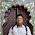Saturday, October 27, 2012

Multiple Choice Questions on Metre Bridge

“If I have seen a little further it is by standing on the shoulders of Giants.”
– Sir Isaac Newton

The metre bridge is basically a Wheatstone bridge, the condition of balance of which is conveniently used in measuring unknown resistances. Today we shall discuss a few questions (MCQ) involving metre bridge:
(1) Resistances R1 and R2 are connected respectively in the left gap and right gap of a metre bridge. If the balance point is located at 55 cm, the ratio R2/R1 is
(a) 4/5
(b) 5/4
(c) 9/11
(d) 11/9
(e) 6/7
The distance of the balance point from the left end of the wire in the metre bridge is 55 cm. Therefore the distance of the balance point from the right end of the wire is 45 cm. Thus we have
R1/R2 = 55/45
Or, R2/R1 = 45/55 = 9/11
(2) A 15 Ω resistance is connected in the left gap and an unknown resistance less than 15 Ω is connected in the right gap of a metre bridge. When the resistances are interchanged, the balance point is found to shift by 20 cm. The unknown resistance is
(a) 5 Ω
(b) 6 Ω
(c) 8 Ω
(d) 10 Ω
(e) 12 Ω

Initially the balance point will be at J1 as indicated in the figure. On interchanging the resistances the balance point will shift to J2 so that the length of the bridge wire between J1 and J2 is 20 cm. The balance points J1 and J2 must be equidistant from the mid point (50 cm mark) of the wire so that J1 is at 60 cm and J2 is at 40 cm.
Therefore we have
R/X =  60/40 = 6/4
Or, 15/X = 6/4
This gives X = 10 Ω
(3) Resistances 4 Ω and 6 Ω are connected across the left gap and right gap respectively of a metre bridge. When a 2 Ω resistance is connected in series with the 4 Ω resistance in the leftt gap, the shift in the balance point is
(a) 10 cm
(b) 15 cm
(c) 20 cm
(d) 25 cm
(e) 30 cm
Initially when the left gap and right gap contain 4 Ω and 6 Ω respectively, the condition of balance is
4/6 = L/(100 L) where L is the balancing length
This gives L = 40 cm.
When a 2 Ω resistance is connected in series with the 4 Ω resistance in the leftt gap, the balancing length becomes 50 cm (since the gaps contain equal resistances).
Therefore the shift in the balance point is (50 cm – 40 cm) = 10 cm.

1 comment:

1.sir question are simple and direct but sometimes it tricks us...